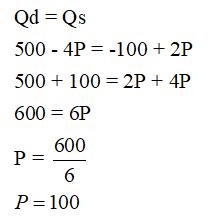# Suppose the market demand curve for cranberry is given by the equation Qd=500-4P, while market supply curve for cranberry is described by the equation Qs=-100+2P where P is the price. Find price.

Question
2 views

Suppose the market demand curve for cranberry is given by the equation Qd=500-4P, while market supply curve for cranberry is described by the equation Qs=-100+2P where P is the price. Find price.

check_circle

star
star
star
star
star
1 Rating
Step 1

Demand function, Qd = 500 - 4P

Supply function, Qs = -100 + 2P

Step 2

Below is the calculation of Price.

At equilibrium point, Qd is equal to Qs.Therefore, price = \$100

...

### Want to see the full answer?

See Solution

#### Want to see this answer and more?

Solutions are written by subject experts who are available 24/7. Questions are typically answered within 1 hour.*

See Solution
*Response times may vary by subject and question.
Tagged in